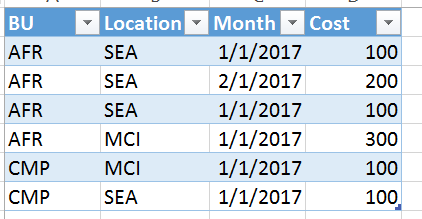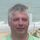cancel
Showing results for
Did you mean:Anonymous
Not applicable

## How to do a running Sum by group in Power Query?

Hi Everyone,

I am trying to do a running sum by group in Power Query (m language).  Thank you.

All solutions I found was to use DAX which I cannot use for my data at this time.

Here is what my data looks like, I would like a running sum of the cost in a new column.

Thank you all.1 ACCEPTED SOLUTIONCommunity Champion

You can use this query (assuming you want to group on "BU"):

```let
Source = Table1,
TableType = Value.Type(Table.AddColumn(Source, "Running Sum", each null, type number)),
#"Grouped Rows" = Table.Group(Source, {"BU"}, {{"AllData", fnAddRunningSum, TableType}}),
#"Expanded AllData" = Table.ExpandTableColumn(#"Grouped Rows", "AllData", {"Location", "Month", "Cost", "Running Sum"}, {"Location", "Month", "Cost", "Running Sum"})
in
#"Expanded AllData"```

`(MyTable as table) as table =>let    Source = Table.Buffer(MyTable),    TableType = Value.Type(Table.AddColumn(Source, "Running Sum", each null, type number)),    Cumulative = List.Skip(List.Accumulate(Source[Cost],{0},(cumulative,cost) => cumulative & {List.Last(cumulative) + cost})),    AddedRunningSum = Table.FromColumns(Table.ToColumns(Source)&{Cumulative},TableType)in    AddedRunningSum`
Specializing in Power Query Formula Language (M)
45 REPLIES 45Frequent Visitor
`Please and if I want the accumulated taking into account the BU and Location columns`Anonymous
Not applicable

These lines:

``````#"Grouped Rows" = Table.Group(Source, {"BU"}, {{"AllData", fnAddRunningSum, TableType}}),
#"Expanded AllData" = Table.ExpandTableColumn(#"Grouped Rows", "AllData", {"Location", "Month", "Cost", "Running Sum"}, {"Location", "Month", "Cost", "Running Sum"})``````

would look like:

``````#"Grouped Rows" = Table.Group(Source, {"BU", "Location"}, {{"AllData", fnAddRunningSum, TableType}}),
#"Expanded AllData" = Table.ExpandTableColumn(#"Grouped Rows", "AllData", {"Month", "Cost", "Running Sum"}, {"Month", "Cost", "Running Sum"})``````Frequent Visitor
```It doesn't work, I already tried
with a similar data I need to calculate the column accumulated by 2 arguments.```Anonymous
Not applicable

Ok.

This is a modified fnAddRunningSum - nothing really important, just made the values column name a variable to untie the internal data structure to the main table from the computation logic in the function:

``````(MyTable as table, values as text) as table =>
let
Source = Table.Buffer(MyTable),
TableType = Value.Type(Table.AddColumn(Source, "Running Sum", each null, type number)),
Cumulative = List.Skip(List.Accumulate(Table.Column(Source, values),{0},(cumulative,cost) => cumulative & {List.Last(cumulative) + Number.From(cost)})),
in

This is the main table and the call to the function above using your sample data:

``````let
Source = Table.FromRows(Json.Document(Binary.Decompress(Binary.FromText("i45WKlTSUUoEYkMDpVgdBBeVZ4xPEsJLxsozwSdpik0yBdVKGNcMlYuNB3JPEgbPBJVrhE1tMqraZKyS5hiSsQA=", BinaryEncoding.Base64), Compression.Deflate)), let _t = ((type nullable text) meta [Serialized.Text = true]) in type table [Est = _t, CICL = _t, ve = _t]),
#"Changed Type" = Table.TransformColumnTypes(Source,{{"Est", type text}, {"CICL", type text}, {"ve", type number}}),

TableType = Value.Type(Table.AddColumn(#"Changed Type", "Running Sum", each null, type number)),
#"Grouped Rows" = Table.Group(Source, {"Est", "CICL"}, {{"AllData", each fnAddRunningSum (_, "ve"), TableType}}),
#"Expanded AllData" = Table.ExpandTableColumn(#"Grouped Rows", "AllData", {"ve", "Running Sum"}, {"ve", "Running Sum"})
in #"Expanded AllData"``````

Please note that the function call has changed slightly as we added the second parameter (value column name) to the function which calculates running totals.

This is the output of the main table/query:

EstCICLveRunning Sum

 q a 10 10 q a 0 10 q a 30 40 q a 0 40 q a 0 40 c a 0 0 c a 40 40 c a 0 40 c a 50 90 c a 0 90 d a 30 30 d a 60 90 d a 0 90 d a 0 90 q b 0 0 q b 40 40 q b 20 60 q b 0 60 c b 40 40 c b 0 40 c b 70 110 c b 0 110

Please let me know if you have any questions.

Kind regards,

JBFrequent Visitor
```I want to thank you for responding to my request, but the code has not worked for me, I don't know what I'm doing wrongAnonymous
Not applicable

I do no think that there is something wrong with the code. It looks Ok.

The error that you get is the "stack overflow", which potentially means that the calculation result does not fit into the variable type.

Could you please try to filter your table (after #"Tipo cambiado" step) to take only 10 top lines to prove that the concept works?

Do you have fairly large numbers in the "Raleo" column?

Thanks,

JBFrequent Visitor
`If you have an email where I can send you the files, I thank you`Anonymous
Not applicable

Hi @Jcarofi,

if this is still not resolved, you can save the sample files to Google Drive, Dropbox or alike and send me a link via private message. I can have a look later today.

Thanks,

JBFrequent Visitor
`As the function is written in the result, you start creating rows by mixing the (ponds) with the (cycles) creating combinations that do not exist`Frequent Visitor

I need a help with de McodeHelper IV

Unbelievable.

Power Bi has invented a billion new functions and formulas but needs 20 lines of code across a query and embedded function to calculate the running total?

Unbelievable.Anonymous
Not applicable

Would be great if you could help me understand how the below statement is working in the code that you provided. I am having a hard time understanding it.

Cumulative = List.Skip(List.Accumulate(Source[SHIPMENT],{0},(cumulative,SHIPMENT) => cumulative & {List.Last(cumulative) + SHIPMENT}))New Member

Hello

To MarcelBeug

This is very good.

Is it possible to give the Field to use (Cost here) as a parameter of the function ?

Thanks a lot

78ChrisNew Member

Hello

I found a solution and give it

```(MyTable as table, MyColumn as text) =>
let
Source = Table.Buffer(MyTable),
TableType = Value.Type(Table.AddColumn(Source, "Cumul", each null, type number)),
Cumulative = List.Skip(List.Accumulate(Table.Column(Source,MyColumn),{0},(cumulative,MyColumn) => cumulative & {List.Last(cumulative) + MyColumn})),
Cumul = Table.FromColumns(Table.ToColumns(Source)&{Cumulative},TableType)
inFrequent Visitor

This is great, though how should I call this function now that it has two arguments?

@78chrisFrequent Visitor

@78chris

Thanks Chris, you posted this code to allow me to select the specific column :

``````(MyTable as table, MyColumn as text) =>
let
Source = Table.Buffer(MyTable),
TableType = Value.Type(Table.AddColumn(Source, "Cumul", each null, type number)),
Cumulative = List.Skip(List.Accumulate(Table.Column(Source,MyColumn),{0},(cumulative,MyColumn) => cumulative & {List.Last(cumulative) + MyColumn})),
Cumul = Table.FromColumns(Table.ToColumns(Source)&{Cumulative},TableType)
in

Below is the original code, which calls this function. What changes need to be made to this to call the function, now that it has two arguments?

``````let
Source = Table1,
TableType = Value.Type(Table.AddColumn(Source, "Running Sum", each null, type number)),
#"Grouped Rows" = Table.Group(Source, {"BU"}, {{"AllData", fnAddRunningSum, TableType}}),
#"Expanded AllData" = Table.ExpandTableColumn(#"Grouped Rows", "AllData", {"Location", "Month", "Cost", "Running Sum"}, {"Location", "Month", "Cost", "Running Sum"})
in
#"Expanded AllData"````````````let
Source = Table1,
TableType = Value.Type(Table.AddColumn(Source, "Running Sum", each null, type number)),
#"Expanded AllData" = Table.ExpandTableColumn(#"Grouped Rows", "AllData", {"Location", "Month", "Cost", "Running Sum"}, {"Location", "Month", "Cost", "Running Sum"})
in
#"Expanded AllData"``````Frequent Visitor

Thanks for the reply @cyongt_bdf. That seems logical, though I'm now getting the following error:

``Expression.Error: There is an unknown identifier. Did you use the [field] shorthand for a _[field] outside of an 'each' expression?``

I've had a search around, but can't find any similar scenarios that generate this same error.

Any thoughts?``````let
Source = Table1,
TableType = Value.Type(Table.AddColumn(Source, "Running Sum", each null, type number)),
#"Expanded AllData" = Table.ExpandTableColumn(#"Grouped Rows", "AllData", {"Location", "Month", "Cost", "Running Sum"}, {"Location", "Month", "Cost", "Running Sum"})
in
#"Expanded AllData"``````Regular Visitor

Hi @cyongt_bdf!

I am having trouble getting this to work. I have to apologize, I'm new to M and power queries custom functions.

When I copied the original table submitted by OP, made it into a source and tried to apply the formulas on it chaning "ColumnHeader" to "Costs". However, I received the following error:

``Expression.Error: The import AddedRunningSum matches no exports. Did you miss a module reference?``

How do I solve this? Additionally, is the name of the function "query" important, i.e. does it need to "fnAddRunningSum" or "AddedRunningSum"?

My actual data is regarding COVID-19 from here https://data.europa.eu/euodp/en/data/dataset/covid-19-coronavirus-data/resource/55e8f966-d5c8-438e-8..., where I'm trying to apply this running sum per country (e.g. "cases" grouped by "countriesAndTerritories").

Thank you very much!